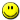/*转载大牛的程序：
先放着-_-nlogn的最大上升子序列长度算法
传统的最大上升子序列采用n2的动态规划算法，就求解一个最大上升子序列的具体序列来说，

对于一个序列a...a[n]，设F[i]表示到第i个数为止的最大上升子序列，我们考虑如这种情况，存

(1) y<x<i;

(2) a[x]<a[y]<a[i];

(3) |F[x]| == |F[y]|;

(4) a[j] < a[x], y < j < x

则此时F[i]应该由F[x]扩展而来，因为可能存在z满足

(1) y<x<z<i;

(2) a[x]<a[z]<a[y]<a[i]

(3) z < min{j | a[j]>a[y], j > x}

则此时用F[x]扩展得到F[i]将长于F[y]扩展得到的子序列。 由此可得出结论，原序列第i个元素之前最长

求解的过程依然是一个动态规划的过程，我们采用一个数组d[k]，来描述到状态i时长度为k的子序列最后一

k = max{a[i]>d[j]} + 1

d[k] = min{d[k], a[i]}

由此我们会发现数组d一个明显的特征，即d是一个单调上升的序列，利用这个特性，我们可以采用二分法来

一下是一段源代码，测试过一个小数据，设计中发现整个算法的难点在于二分法查找的设计。
*/#include <cstdio>#include
<cstdlib>#include
<climits>#include
<iostream>using namespace std;#define MAX
1000int a[MAX];int d[MAX];int max_subsequence (const int size ){int n;d[ n
= 0 ] = a[ 0 ];for ( int i = 1; i < size; i++ )if ( d[ n ] < a[ i ] )d[
++n ] = a[ i ];else if ( d[ 0 ] > a[ i ] )d[
0 ] = a[ i ];else{int left = 0right = n, mid = n / 2, key = a[ i ];while ( left < right ){if ( d[ mid ] == key ){mid--;break;}if ( d[ mid ] > key ){right = mid;mid = ( left + right ) / 2;}else if ( mid > left ){left = mid;mid = ( left + right ) / 2;}elsebreak;}if ( d[ mid + 1 ] > key )d[
mid + 1 ] = key;}return n
+ 1;}int main ( ){int n;scanf (
"%d"&n );for ( int i = 0; i < n; i++ )scanf (
"%d"&a[ i ] );printf (
"%d\n", max_subsequence ( n ) );//  system ( "pause" );return
0;}